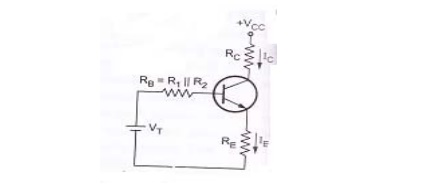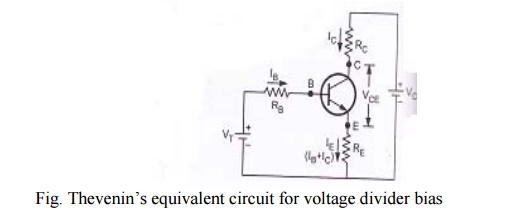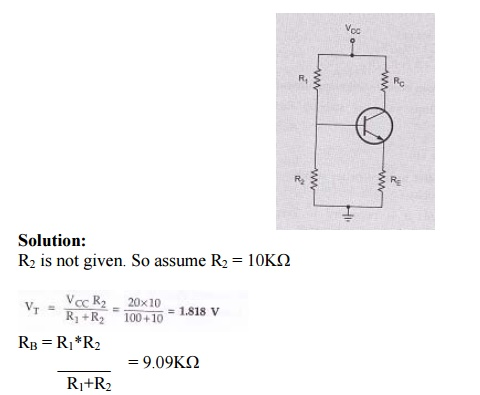Home | | Electronic Circuits I | Voltage divider bias circuit

# Voltage divider bias circuit

Figure shows the voltage divider bias circuit. In this, biasing is provided by three resistors R1, R2 and RE.

Voltage divider bias circuit:Figure shows the voltage divider bias circuit. In this, biasing is provided by three resistors R1, R2 and RE. The resistors R1& R2 act as a potential divider giving a fixed voltage to base. If collector current increases due to change in temperature or change in β, emitter current IE also increases and voltage drop across RE increases thus reducing the voltage difference between base and emitter. Due to reduction in base emitter voltage, base current and collector current reduces. So we can say that negative feedback exists in emitter bias circuit. This reduction in collector current compensates for the original change in IC.

Circuit analysis:

Base circuit:Fig. Base circuit

Let us consider the base circuit as shown in above figure. Voltage across R2 is base voltage VB. Applying voltage divider rule to find VBCollector circuit:Let us consider the collector circuit as shown in above figure. Voltage across RE can be obtained as,Simplified circuit of voltage divider biasFig.Thevenin’s equivalent circuit for voltage divider bias

From above figure, R1 and R2 are replaced by RB and VT.

Where RB is the parallel combination of R=1 and R2

VT is the thevenin’s voltageProblem 1:

For the given circuit β=100 for silicon transistor. Calculate VCE and ICProblem 2:

For the given figure find Q point with VCC = 15V, VBE = 0.7V and β = 100.Stability factor for voltage divider bias:

Stability factor S:For determining stability factor S for voltage divider bias, consider the equivalent circuit. Thevenin’s voltage is given by,R1, R2 are replaced by RB which is the parallel combination of R1 and R2.Apply KVL to base circuit,Differentiating with respect to IC and considering VBE to be independent of IC,From above equation, the following points are observed.

1. The ratio RB /RE controls value of stability factor S. If RB/RE<< 1 then it is reduced to S = (1+β). 1/ (1+β) = 1

Practically RB/RE not equal to zero. But to have better stability factor S , we have to keep ratio RB/RE as small as possible.

2.     To keep RB/RE small, it is necessary to keep RB small. Due to small value of R1 and R2, potential divider circuit will draw more current from VCC reducing the life of the battery. Another important aspect is that reducing RB will reduce input impedance of the circuit, since RB comes in parallel with the input. This reduction of input impedance in amplifier circuit is not desirable and hence RB cannot be made very small.

3.     Emitter resistance RE is another parameter, it is used to decrease the ratio RB/RE. Drop across RC will reduce. This shifts the operating point Q which is not desirable and hence there is limit for increasing RE.

While designing voltage divider bias circuit, the following conditions are to be satisfied,

S   – Small

RB    - Reasonably small

RE     - Not very large

4.     If ratio RB/RE  is fixed, S increases with β. So stability decreases with increasing β.

5.     Stability factor S is essentially independent of β for small value of S.

Substituting the differentiation value of IB /IC,Problem 1:

For the given circuit, VCC = 20V, RC = 2KΩ, β = 50, VBE = 0.2V, R1 = 100KΩ, RE = 100Ω. Calculate IB, VCE, IC and stability factor S.Study Material, Lecturing Notes, Assignment, Reference, Wiki description explanation, brief detail
Electronic Circuits : Biasing of Discrete BJT and MOSFET : Voltage divider bias circuit |